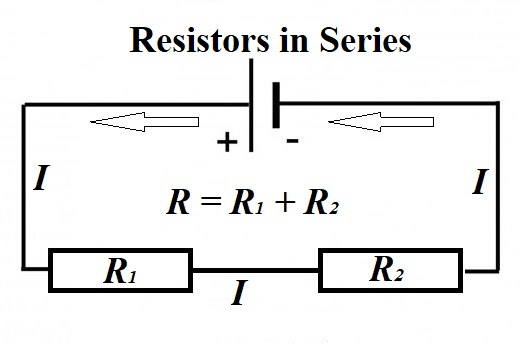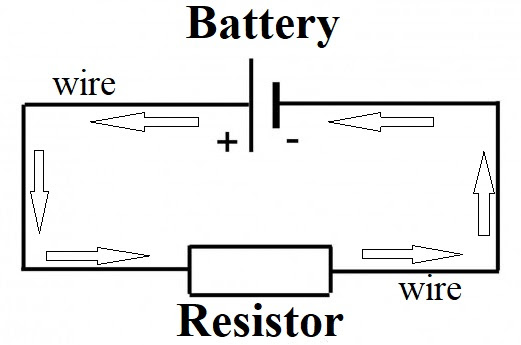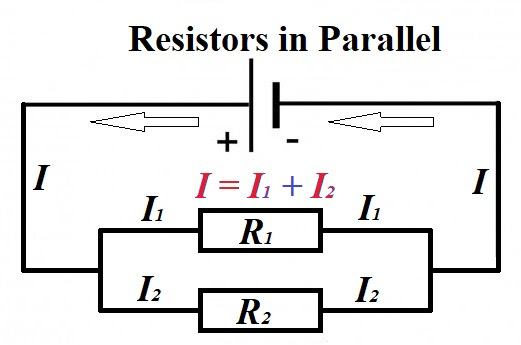## Sunday, March 29, 2020

### Unizor - Physics4Teens - Electromagnetism - Votage Drop

Notes to a video lecture on http://www.unizor.com

Direct Current - Voltage Drop

Imagine a waterfall going down the rocks. As it hits each rock, it loses some of its potential energy.
Analogously, consider several radiators in a series connected to a boiler that supplies hot water for them. As the water goes from one radiator to another, it cools down, losing its heat energy.
The same happens with electrons, as they go along the circuit and meet one resistance after another. Going through each of them, they lose their energy.

Consider the following circuitAssume, we know how to measure the difference in potential (voltage) between any two points on this circuit. Measuring the voltage between the terminals of the battery gives some value U.
Measuring the voltage between the positive terminal of the battery (the longer thin line with a plus sign) and the point in-between the resistors gives some other value U1.
Measuring the voltage between the negative terminal of the battery (the shorter thick line with a minus sign) and the point in-between the resistors gives yet other value U2.

Notice that the amount of electricity going through this circuit per unit of time (electric current or amperage) is the same everywhere since it's a closed loop and equals I.

Now let's apply the Ohm's Law to an entire circuit, keeping in mind that the voltage between the terminals of the battery is U and the total resistance of an entire circuit is R=R1+R2:
I = U / R = U / (R1+R2)

Applying the Ohm's Law to a segment of a circuit that includes only resistor R1 and knowing the voltage on its two ends U1, we obtain
I = U1 / R1

Equating two different expressions for the electric current I, we can find the voltage U1:
I = U / (R1+R2) = U1 / R1
from which the value of U1 is
U1 = U·R1 / (R1+R2)

Let's do the same calculation for the second resistor.
I = U / (R1+R2) = U2 / R2
from which the value of U2 is
U2 = U·R2 / (R1+R2)

Notice that U1 + U2 = U.
Indeed,
U·R1 / (R1+R2) +
+ U·R2 / (R1+R2) =
= U·(R1+R2/ (R1+R2) = U

Completely analogous calculations can be provided with three or more resistors connected in a series.
So, the difference in electric potential or voltage drop between the terminals of a battery U is split between the voltage drops on each resistor in a series.

## Saturday, March 28, 2020

### Unizor - Physics4Teens - Electromagnetism - Ohm's Law

Notes to a video lecture on http://www.unizor.com

Direct Current - Ohm's Law

Simply speaking, experiment shows that the current in a conductor (amperage) is proportional to a difference in potentials (voltage) on its ends. This is the Ohm's Law.

Obviously, some physical explanation of this law is appropriate, and this is what this lecture is about.

Electric current is the flow of electrons inside a conductor. Just as a reminder, the traditionally defined direction of the current is opposite to the direction of the flow of electrons.

Even without any electric field electrons inside a conductor are not exactly spin each around its own nucleus, staying on the orbit forever. Some of them, especially those on the outer orbit, can tear off their orbit, jump to another nucleus' orbit, then going somewhere else etc., thus creating certain chaotic environment.
When an electric field is present, the chaotic movement of electrons becomes directional to a degree, depending on the strength of the field, thus creating a flow of electrons - an electric current.

This flow of electrons occurs when there is an excess of electrons on one end and deficiency (or less of an excess) of electrons on the other end, which creates an electric field inside a conductor that forces some light electrons to leave their atoms and move, while heavy nuclei with remaining electrons stay put.
Electrons are repelled from the end, where there is excess of them and attracted (or repelled less) from the other end.

On their way from one end of a conductor to another these electrons must go through a maze of atoms, many of which have lost some electrons because of the electric field and, therefore, are positively charged, attracting negative electrons in an attempt to compensate lost electrons. Some succeed by capturing free electrons, some not, some lose more electrons in their chaotic but directional movement.

The flow of electrons inside a conductor can be compared with a waterfall from the high level, where potential energy relative to gravitational field of the Earth is greater to a lower level with lesser potential energy.

As water falling down the waterfall, hitting all the stones on its way, electrons hit atoms and lose some of their energy, some of them get absorbed by atoms and don't reach the other end of a conductor, thus diminishing the flow.

The very important aspect in this movement of electrons is the properties of the material a conductor is made of.

Different atoms of different materials have different characteristics of capturing and releasing electrons, when subjected to the forces of an electric field. However, certain common laws of conductivity can be logically explained and used in practical applications.

Ohm's Law

Stronger electric field produced by a greater difference in electric potential (voltage U) between the ends of a conductor should cause more intense movement of electrons, greater number of electrons going from one end of a conductor and reaching the other end per unit of time (amperage I). This qualitative property is perfectly understood. Mathematically speaking, I is a monotonically increasing function of U.

It was an experimental fact that this monotonic function to a very high precision is linear:
I = σ·U
where σ is called a conductivity of a conductor.
In more common cases this law is written not in terms of conductivity, but in terms of resistivity:
I = U / R
where R is called a resistance of a conductor.

In most cases we will use the Ohm's Law in that last form, using a resistance as the characteristic of a conductor.

Resistance

In practical life we use some conductors with a very low resistance, like copper wire, to connect some electrical appliance, like a regular incandescent lamp bulb, to a source of electric power. In this arrangement we usually consider wiring as having no resistance and concentrate on the properties of an appliance as the one with the electrical resistance R.

Schematically, resistor is pictured as a rectangle or a zigzag line connected by straight lines of wires to a source of electric powerArrows on this picture show the traditionally defined direction of electricity from positive to negative terminal of the source of electric power - opposite to a direction of electrons' movement.

Consider an extreme case when the resistor is non-conductive, like there is only vacuum in between its two ends. Symbolically, it's equivalent to R=∞. The Ohm's Law in this case results in I=U/R=0, which means that the circuit is broken and there is no current in it.

In an opposite extreme case with R=0 we have I=U/R=∞, which is the so called "short".
In practical life it happens when you detach two wires coming to a lamp and connect them. Without the bulb, which has some significant resistance, the electric current in a circuit would almost instantaneously grow very high and you might get electrocuted.

Let's use an incandescent lamp as an example of a resistor. More precisely, the resistor is a tangent spiral (filament) inside the lamp.

Consider what happens with the resistance of this tangent spiral if we will make it longer.
Obviously, the electrons will have to go through more obstacles on their way from one end of a spiral to another, which will slow their movement more than when a spiral was shorter. It's logical to expect that doubling the length of a resistor will double its resistance, and it is confirmed by experiments.
In general, for linearly-shaped resistors their resistance should be proportional to the length.

The immediate consequence of this consideration is that two resistors, sequentially connected one after another in a series, will have a combined resistance equal to a sum of resistance of each one.Now, instead of making a spiral longer, let's make it thicker. Electrons will have more space to move, more freedom of direction and more electrons can travel across the spiral per unit of time. Doubling the thickness of a spiral is similar to doubling the width of a highway with more cars moving on it per unit of time. So, the resistance of a thicker spiral will decrease by the factor of increased cross-section area of a tangent spiral.
In general, for linearly-shaped resistors their resistance should be inversely proportional to the cross-section area.

Increasing the thickness of a spiral is logically equivalent to using two spirals connected parallel to each other, as on this circuit diagram.The number of electrons per unit of time (electric current or amperage) coming from the common wire is split into two parallel flows, and all the electrons passing through a common part of a circuit per unit of time should be equal to a sum of numbers of electrons passing through each of the parallel segments.
The immediate consequence of this consideration is that two resistors, connected parallel to each other, will result in the electric current in a common wire to be a sum of electric currents in each one of them.
I = I1 + I2

Now let's apply the Ohm's Law to an entire circuit, and each of two parallel branches, keeping in mind that the voltage between the terminals of the battery is U, the same as the voltage between the ends of each resistor, and the resistance of each branch is known as R1 and R2.

Assuming the total resistance of two parallel branches is R, the Ohm's Law gives
I = U / R

Applying the same principle to one branch with resistor R1, we have
I1 = U / R1

The same for another branch:
I2 = U / R2

Now the original equation about a current in the common segment of a wire equaled to a sum of currents in two branches looks like
/ R = U / R1 + U / R2
or
1/R = 1/R1 + 1/R2

From the last equation follows the expression for a combined resistance of two resistors installed parallel to each other
R = 1 / (1/R1 + 1/R2)
This formula looks more cumbersome and the one for 1/R above is more commonly occurs.

Instead of resistance, it can be expressed in terms of conductivity of an entire assembly σ=1/R of two parallel resistors with conductivity σ1=1/R1 and σ2=1/R2:
σ = σ1 + σ2

For two identical resistors of resistance r each the combined resistance R would be
1/R = 1/r + 1/r = 2/r
R = r/2

More generally, we can derive the resistance of n identical resistors of resistance r each connected parallel to each other. In this case the electric current I in the common wire in split into n identical flows, each having an amperage of I/n. From the Ohm's Law the voltage at the ends of each resistor should be U=I·r/n, from which follows that R=r/n is the resistance of an entire assembly of n identical resistors with resistance r each.

Example

Consider a circuit with resistors R1 and R2 connected parallel to each other and resistor R3 installed after both of them in a series.
What would be the resistance R of a combined assembly of these three resistors?

First, determine the resistance of two parallel resistors as a unit.
R12 = 1 / (1/R1 + 1/R2)
This this unit of two parallel resistors with resistance R12 is in a series with resistor R3, their combined resistance is the sum of their individual resistances
R = R12 + R3 = [/ (1/R1 + 1/R2)] + R3

Resistance Units
of Measurement

The Ohm's Law allows to easily establish the units of measurement for the resistance of any component in an electric circuit - ohms Ω.
From the original form of the Ohm's Law
I = U / R
follows that
R = U / I

This allows to establish a unit of measurement for resistance of any component of an electric circuit.
If the difference in potential (voltage) between one end of such a component and another is 1V (volt) and the electric current going through it is 1A (ampere), this component by definition has a resistance of 1Ω (ohm).

Consequently, the resistance of a component in an electric circuit with the current going through it I (ampere) with voltage on its end (volt) equals to / I (ohm).

## Wednesday, March 11, 2020

### Unizor - Physics4Teens - Electromagnetism - Electric Current - Speed of ...

Notes to a video lecture on http://www.unizor.com

Speed of Electrons

Electric current is a movement of electrons. We know from experience that, when we turn on the switch, the lights in the room are lit practically immediately. Does it mean that electrons from one terminal of a switch go to the light fixture and back to another terminal of a switch that fast?
No.

Let's calculate the real speed of electrons, first, theoretically and then in some practical case.

Assume, the amperage of the electric current going through a wire, that is the number of coulombs of electric charge going through a wire per second, is I, and the wire has cross-section area A.
Assume further that we know all the physical characteristics of a material our wire is made of, which will be introduced as needed.

Based on this information, our plan is to determine the number of electrons going through the wire per unit of time and, knowing the density of electrons per linear unit of length in the wire, determine the linear speed of these electrons.

Obviously, to determine the linear density of electrons, we will need physical characteristics of a wire.

The number of electrons going through a wire per unit of time is easily determined from the amperage I. Since I represents the number of coulombs of electric charge going through a wire per second, we just have to divide this by the charge of a single electron in coulombs e=−1.60217646·10−19C.
So, the number of electrons going through a wire per second is
Ne = I / e.

Now we will determine the linear density of electrons in the wire.

First of all, we have to know how many active electrons in an atom of material our wire is made of participate in the transfer of electric charge, because not all electrons of each atom are freely moving in the electric field, but only those on the outer orbit. Let's assume, this number is ne.
Using this number, we convert the number of electrons participating in the transfer of electric charge Ne into the number of atoms Natoms in that part of a wire occupied by all electrons transferring the given charge per second I.
Natoms = Ne / ne = I / (ne·e).

Next, from the number of atoms we will find their mass and, using the density of wire material, the volume.
Dividing the volume by a cross-section of a wire, we will get the length of a segment of wire occupied by those electrons transferring charge per second, which is the speed of electrons or drift.

Knowing the number of atoms, to get to their mass, we will use the Avogadro number
NA=6.02214076·1023
that represents the number of particles in one mole of a substance. One mole of material our wire is made of is the number of grams equal to its atomic mass ma, known for any material used for a wire.
If NA atoms have mass of ma gram, Natoms have total mass
Matoms = ma · Natoms / NA =
= ma·I / (ne·e·NA)
.

Knowing the total mass Matoms of all atoms that contain all electrons traveling through a wire per second, we calculate the volume Vatoms by using the density ρ of a material the wire is made of.
Vatoms = Matoms / ρ =
= ma·I / (ne·e·NA·ρ)

Dividing the volume Vatoms by the cross-section area of a wire, we will get the length of the wire L occupied by electrons traveling through it in one second
L = Vatoms / A =
= ma·I / (ne·e·NA·ρ·A)

where
ma - atomic mass of wire's material (assuming it's one atom molecules, like copper)
I - electric current - amperage in the wire
ne - number of active electrons in each atom of wire's material that participate in the transfer of electric charge
e - electric charge of one electron
NA - number of atoms in 1 mol of conducting material - Avogadro Number
ρ - density of wire's material
A - cross-section area of a wire

The above formula represents the length of a wire occupied by all active electrons traveling through it during one second, which is the speed of movement of electrons making up an electric current, called drift.

Let get to practical examples.

Assume, the voltage or the difference of electric potential E between two ends of a copper wire is maintained at 110V (standard voltage for apartments in the USA).
This wire connects a lamp that consumes 120W of electric power P (or wattage).
Let the cross-section area of a wire be 3 mm².

First of all, let's calculate the amount of electricity moving through the wire per unit of time - amperage I.
As we know, the amperage I, multiplied by voltage E, is the electric power P (wattage).
Therefore,
E = 110V
P = 120W
I = P/E = 60W/110V ≅ 1.09A

The atomic number of copper is Z=29. It means, the atom of copper has 29 protons in the nucleus and 29 electrons orbiting a nucleus. These 29 electrons are in four orbits: 2+8+18+1.
The outer orbit has only one electron that participates in the movement of electric charge, so the number of active electrons in an atom of copper is
ne=1.

The nucleus of an atom of copper has 29 protons and 34 or 36 neutrons, its atomic mass is
ma = 63.546g/mol

The electric charge of one electron is
e = −1.60217646·10−19C.

NA=6.02214076·1023

Density of copper is
ρ = 8.96 g/cm³ = 0.00896 g/mm³

Cross-section area of a wire is
A = 3 mm²

Using the formula above with values listed, we obtain
L = ma·I / (ne·e·NA·ρ·A)
we get
L ≅ 0.0267 mm/sec
This is the speed of electrons traveling along a copper wire in this case.
Pretty slow!